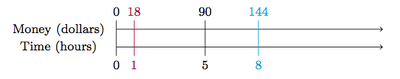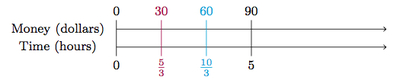# Gianna's Job

Alignments to Content Standards: 6.RP.A.3.a 6.RP.A.3.b 6.RP.A.3

Gianna is paid \$90 for 5 hours of work. 1. At this rate, how much would Gianna make for 8 hours of work? 2. At this rate, how long would Gianna have to work to make \$60?

## IM Commentary

The purpose of this task is to apply reasoning about ratios to solve a rate problem. This problem introduces a rate whose units are dollars per hour of work. Using this information, students need to make two separate calculations, one with units of dollars and the other with units of hours. Multiple approaches are possible, including

• making a ratio table,
• making a double number line,
• finding a unit rate.

Each of these methods is important and the teacher may wish provide extra instructions to make sure that each method is represented by student work. This task would be ideal for group work: different groups could be directed to solve the problem in different ways if desired.

The table and number line methods are very closely related, differing mainly in how the information is laid out. In the solutions we used different strategies with these methods but it could also be interesting for the teacher to highlight the similarities by using the same approach in both. The unit rate approach can be closely linked with the table and number line depending on how these are implemented: for example, the number line method for solving part (a) below corresponds precisely to ''finding the unit rate.''

This task is based upon Exercise 18 from section 7.2 of Sybilla Beckmann's Mathematics for Elementary School Teachers, Pearson, 2014. Ideas from the commentary and solution have been gathered from a fall 2013 class of prospective teachers at the University of New Mexico.

The Standards for Mathematical Practice focus on the nature of the learning experiences by attending to the thinking processes and habits of mind that students need to develop in order to attain a deep and flexible understanding of mathematics. Certain tasks lend themselves to the demonstration of specific practices by students. The practices that are observable during exploration of a task depend on how instruction unfolds in the classroom. While it is possible that tasks may be connected to several practices, the commentary will spotlight one practice connection in depth. Possible secondary practice connections may be discussed but not in the same degree of detail.

When recognizing ratios, rates, and proportional relationships, students are actively engaged in Mathematical Practice Standard 7, Look for and make use of structure. In this task, students use the structure of ratios to solve a rate problem.  They are given a rate whose units are dollars per hour and are asked to answer questions involving units of dollars and units of hours. The teacher may build on the structure of ratios by guiding students to construct ratio tables, double number lines or finding the unit rate and using that to solve the task.  This task not only allows students to recognize structure, but apply the structure in a real world situation.

## Solutions

Solution: 1 Making a table

1. This method uses a ratio table:

Time Worked (hours) Gianna's Earnings (dollars)
5 90
10 180
20 360
40 720
8 144

The first row is the given information and to get to the second row we multiply both entries of the first row by 2. To get from the second to the third row of the table we multiply by 2 again. From the third to the fourth for we multiply by 2 for a third time. Now 40 hours can be divided by 5 to give 8 hours so this is the last step. There are many other possible ways to arrive at the answer with a table. For example, since $$8 = \left(\frac{8}{5}\right) \times 5$$ we could move from the first row to the last in one step, multiplying the first row by $\frac{8}{5}$.

2. We again make a table and this time the goal is to get \$60 in the earnings column and find out how many hours it takes for Gianna to earn this amount of money. We see that 60 is not a factor of 90 so we can not get to 60 directly by dividing by a whole number. But 60 is a factor of 180 which is 2$\times$90 so we use this: Time Worked (hours) Gianna's Earnings (dollars) 5 90 10 180$\frac{10}{3}$60 It takes Gianna$\frac{10}{3}$hours or 3 hours and 20 minutes to make \$60.

Solution: 2 Making a double number line

1. We are given that Gianna makes \$90 in 5 hours. We can plot this information on a double number line, with money plotted on one line and time on the other:The goal is to use the information given to work out what dollar amount will go along with 8 hours. One way to do this would be to work out the hourly wage and then multiply by 8. This is shown below with the first step drawn in purple and the second step in blue:To find the hourly wage we have to divide the number of given hours by 5 and so we also divide the wages by 5. Next, to find the wages for 8 hours we multiply the hourly wage by 8. There are many other alternatives. The quickest method would be to multiply the given values of money and time by$\frac{8}{5}$. 2. To find how long Gianna has to work to make \$60 notice that \$60 is$\frac{2}{3}$of \$90. So we can first take one third of the the given values (in purple below) and then double these new values (in blue):It takes Gianna $\frac{10}{3}$ hours or 3 and a third hours to earn \$60. Solution: 3 Using a unit rate 1. In order to find out how much Gianna makes in 8 hours, we can first find her hourly rate and then multiply by 8. Since Gianna makes \$90 in 5 hours she will make \$90$\div$5 in 1 hour. This means that Gianna makes \$18 per hour. So in 8 hours she will make 8 $\times$ \$18 = \$144.

2. To find out how long it takes Gianna to make \$60 we can find out how long it take her to make \$1 and then multiply by 60. Since Gianna makes \$90 in 5 hours she will make \$1 in 5 $\div$ 90 hours. This is $\frac{1}{18}$ of an hour. Since Gianna makes \$1 in$\frac{1}{18}$of an hour she will make \$60 in $\frac{60}{18}$ hours. This is three and a third hours.

Although the solutions to (a) and (b) are conceptually similar, (a) feels more natural because we use the units of dollars per hour frequently when thinking of wages. For part (b), we use the units of hours per dollar which feel less familiar.